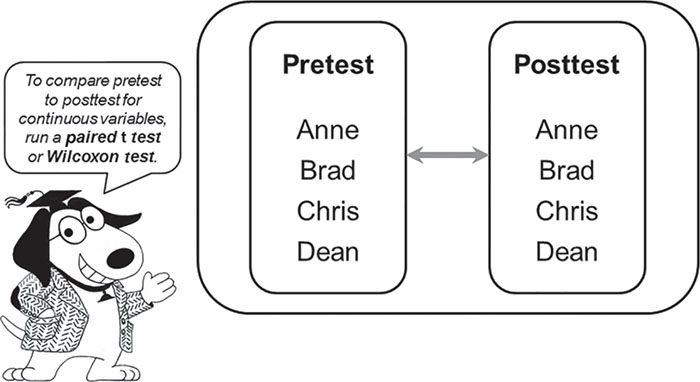What statistical test should I use for this kind of data? How do I set up the data? What parameters should I specify when ordering the test? How do I interpret the results? Herschel Knapp’s friendly and approachable guide to real-world statistics answers these questions. Intermediate Statistics Using SPSS is not about abstract statistical theory or the derivation or memorization of statistical formulas–it is about applied statistics. With jargon-free language and clear processing instructions, this text covers the most common statistical functions–from basic to more advanced. Practical exercises at the conclusion of each chapter offer students an opportunity to process viable data sets, write cohesive abstracts in APA style, and build a thorough comprehension of the statistical process. Students will learn by doing with this truly practical approach to statistics. Free downloadable tutorial videos provide an overview of each statistical method!

# Paired t Test and Wilcoxon Test

### Paired t Test and Wilcoxon TestThings never happen the same way twice.

—C. S. Lewis

### Learning Objectives

Upon completing this chapter, you will be able to do the following:

• Determine when it is appropriate to run a paired t test.
• Verify that the data meet the criteria for paired t test processing: normality of differences.
• Order a paired t test.
• Interpret test results.
• Resolve the hypotheses.
• Write an appropriate abstract.
• Calculate and document the Δ% formula.
• Know when and how to run and interpret the Wilcoxon test.

### When to Use This Statistic### Guidelines for Selecting the Paired t Test and Wilcoxon Tests

Overview: This statistic detects if a measurement changes from one time point to another time point.

Variables: This statistic requires two continuous variables for each record: (1) the score at an initial time point and ...

•••